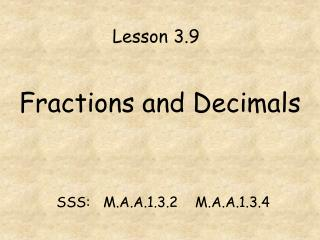DownloadDownload PresentationFractions and Decimals

Fractions and Decimals

Télécharger la présentationFractions and Decimals

- - - - - - - - - - - - - - - - - - - - - - - - - - - E N D - - - - - - - - - - - - - - - - - - - - - - - - - - -
Presentation Transcript

1. Lesson 3.9 Fractions and Decimals SSS: M.A.A.1.3.2 M.A.A.1.3.4

2. Warm Up Write as an Improper Fraction: Write as a Mixed Number (Simplest Form): 1) = 3) = 4) 2) = = *Remember: Whole number: # of times denominator goes into numerator. Numerator = the remainder after dividing Denominator stays the same *Remember: (around the world with TP) Numerator = bottom Times whole Plus top Denominator stays the same

3. To change a fraction to a decimal, divide the numerator by the denominator. Example: = = .625 *Remember: The top number ALWAYS gets “caught” in the division box. Also: when dividing don’t forget your decimal, line up your answer, and add zeros as needed to bring down.

4. Here is another example and a few to try.*remember- divide the numerator by the denominator. Example: Now you try a few: 1. 2. 3.

5. A decimal that stops or “terminates” is a terminating decimal If the same block of digits in a decimal repeats without end, the decimal is a repeating decimal Show a repeating block by putting a bar over the repeating digits. = = Example:

6. Is this a repeating decimal???? 3.03003000300003… Try a few: (.18181818…) 1) = 2) = (.3333333…) 3) = (.5833333…)

7. Changing a Decimal into a fraction • First write the number on the left of the decimal as the whole number. • Next, write the decimal amount as a • numerator. • Then for the denominator write the • number 1 and add a zero for each place • value to the right of the decimal. • Finally: simplify the fraction if necessary. 2.48 Example: = =

8. Try a few… Change the following decimals into fractions: *Don’t forget to simplify 1) 4.7 2) 9.75 3) 1.36

9. Comparing Fractions and Decimals • To compare values: • First, change ALL to either decimals or fractions. • Then, compare and write your list • according to directions…least to greatest • or greatest to least. • Finally, write the list in the original form • that it was in the problem.

10. Here is one to try…Put the following in order from least to greatest 3.23, , 5.75, , 5.015 3.23, , 5.015, , 5.75